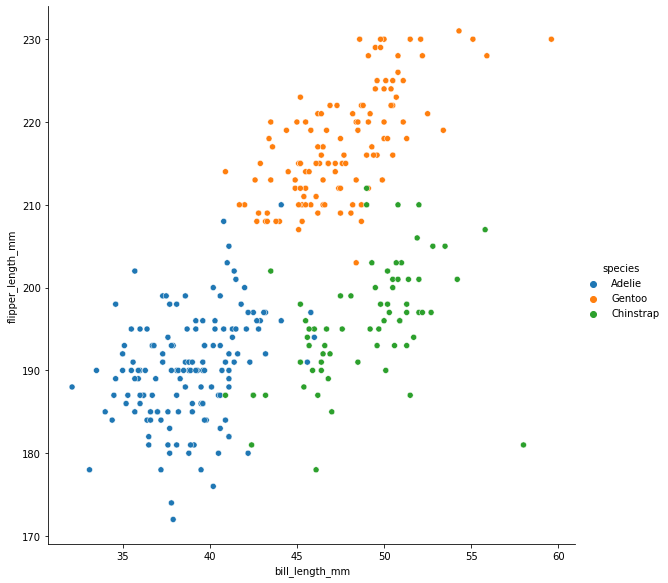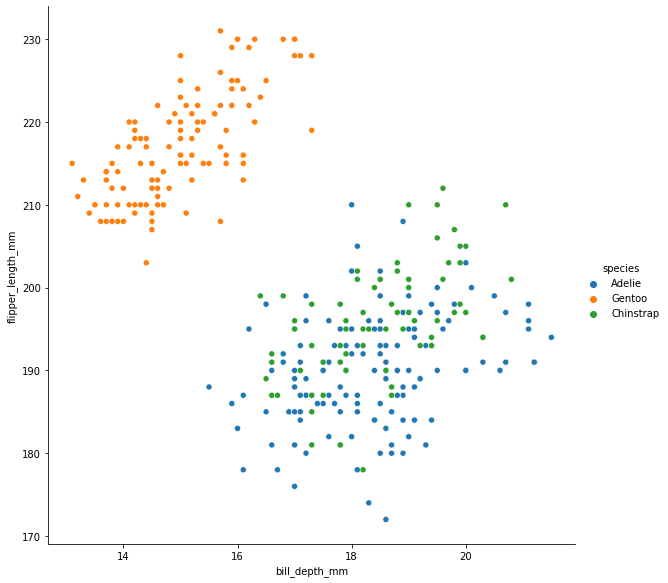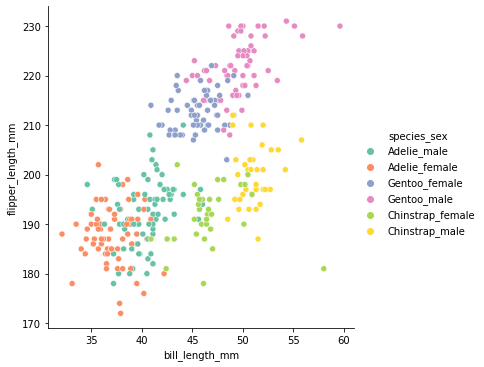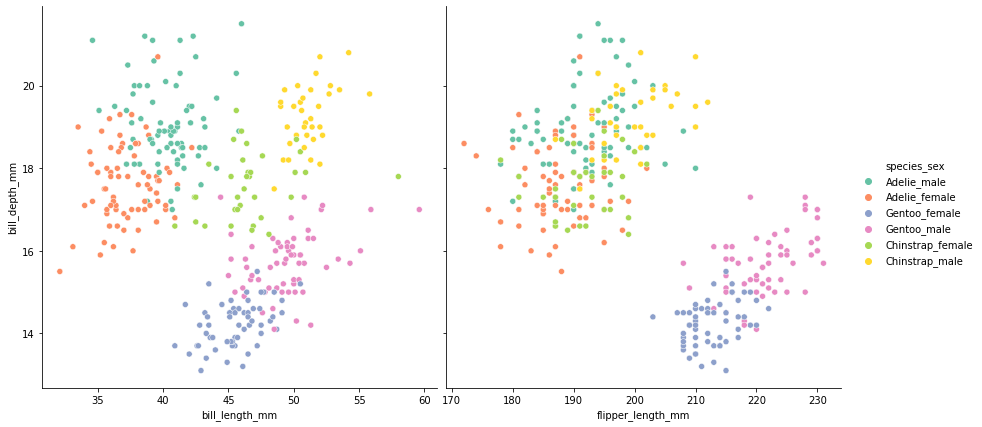# Introduction¶

## What is machine learning?¶

Machine learning is programming by example. There are a couple of different categories of problems that fall into the machine learning category. The easiest to understand is probably classification, so we'll concentrate on that initially.

### Classification¶

This is where we want to take a data point and assign it to one of a number of different categories. This is probably the easist type of problem to understand. For example:

• given the blood results of a patient, decide whether or not they have a particular disease
• given the measurements of the wings of a butterfly, decide which species it belongs to
• given the sequence of a gene, decide whether it comes from a bacteria or a eukaryote

For most classification problems, it's best to think of the output as a prediction, that could be checked given sufficient resources. For example, if our patient is predicted to have the disease, we might confirm it with a test. Or we could take our buttefly and show it to an expert who could tell us which species it belongs to. Or we could take our gene sequence and do a similarity search against a large database of bacterial genes to check it.

### Explicit rules vs. learned rules¶

Imagine a trivial classification problem: determining whether a biological sequence is DNA or protein. If we had to write a bit of code to do the job, we might take an approach like this:

In :
def classify_sequence(mystery_sequence):

a_count = mystery_sequence.count('A')
t_count = mystery_sequence.count('T')
g_count = mystery_sequence.count('G')
c_count = mystery_sequence.count('C')

bases_count = a_count + t_count + g_count + c_count

# guess that a sequence is DNA if more than 90% of the characters are bases
if bases_count / len(mystery_sequence) > 0.9:
return 'DNA'
else:
return 'Protein'


We can easily test this out on a few examples:

In :
for seq in ['ATCGATCGTACGTACGATCGTACTGAT', 'MFADRWLFSTNHKDIGTLYLLFGAWAG']:
print(seq, classify_sequence(seq))

ATCGATCGTACGTACGATCGTACTGAT DNA


The function isn't foolproof - we may occasionally run into a protein sequence that's rich in alanine, threonine, glycine and cystine (giving us lots of ATGC characters), but we can imagine it working most of the time. The most important part of the function is the rule implemented by the if, which says that if most of the characters in a sequence are A, T, G or C, then it's probably DNA. This is typical of many programs: we come up with the rules we want to implement, then figure out how to translate them into code.

A machine learning approach to solving this problem would require a different strategy. Rather than coming up with the rules ourselves, we would instead collect a bunch of DNA and protein sequences, generate the character counts, then pass those examples to the computer and have it figure out the rules based on the examples. Much of the complexity in machine learning revolves around deciding which features of the examples we want to use (in this case, the character counts) and the algorithm that the computer uses to figure out and represent the rules.

## Classifying penguin species¶

To illustrate this idea of programming by example, we'll use a real life dataset. The Palmer Penguins dataset has been assembled specifically as an example to be used for learning data exploration skills.

We can start by using pandas to load the dataset directly from the source URL:

You'll need to know a bit of pandas in order to follow along with this example - the first few chapters of the Biological Data Exploration book will give you all the information you need. To keep things simple, we'll just load the columns that we're interested in, and drop any rows with missing data:

In :
import pandas as pd

df = (
"https://raw.githubusercontent.com/allisonhorst/palmerpenguins/master/inst/extdata/penguins.csv",
)
.dropna() # missing data will confuse things
)

df[['flipper_length_mm', 'bill_length_mm', 'species']] # selecting columns rather than passing usecols allows us to reorder them

Out:
flipper_length_mm bill_length_mm species
... ... ... ...
339 207.0 55.8 Chinstrap
340 202.0 43.5 Chinstrap
341 193.0 49.6 Chinstrap
342 210.0 50.8 Chinstrap
343 198.0 50.2 Chinstrap

333 rows × 3 columns

The data are hopefully fairly straightforward to understand. We have a sample of 342 penguins of three different species:

In :
df['species'].value_counts()

Out:
Adelie       146
Gentoo       119
Chinstrap     68
Name: species, dtype: int64

For each penguin we know the species and two measurements: bill length and flipper length (both in milimeters). Our challenge is to write a program that will take the bill and flipper length of a penguin and predict its species. We can imagine this being useful in the field - perhaps we are studying closely related species that are hard to distinguish, but easy to measure. So having such a classification tool might allow us to use non-expert volunteers to gather the measurements, then figure out the matching species later on.

Before we try the programming-by-example approach of machine learning, let's see how we might solve the problem using explicit rules, as we did for the sequence classification example. It will be easier if we make a quick visualization to see how the two measurements differ between species. For this we will use my favourite charting tool: seaborn (chapters 6 and 7 of Biological data exploration cover the tools used here).

A simple scatter plot gives a nice graphical overview:

In :
import seaborn as sns

sns.relplot(
data = df,
x = 'bill_length_mm',
y = 'flipper_length_mm',
hue = 'species',
height=8,
)

Out:
<seaborn.axisgrid.FacetGrid at 0x7f02ca2cd580>It's hopefully clear from this figure that coming up with a rule to identify Gentoo penguins (the orange points) should be quite easy. We can separate them out well just by looking at flipper length - nearly all of the penguins with flippers longer than 205mm are Gentoo. Distinguishing between the two other species will clearly be a bit trickier. If we concentrate on the blue and green points in the bottom half of the chart, we see that there's a lot of overlap in the y axis (flipper length). Happily, there's a clear separation in bill length - most of the points to the left of 45mm are Adelie (blue) and most of the points to the right are Chinstrap (green).

So let's go ahead and implement those rules in a function:

In :
# there are many different ways to implement the rules, this seems clearest
def classify_penguin(bill_length, flipper_length):
if flipper_length > 205:
return 'Gentoo'
elif flipper_length <= 205 and bill_length > 45:
return 'Chinstrap'
else:


How can we test this function to see how good a job it does of correctly identifying penguin species? The simplest thing is to run it on each of the rows of our existing dataframe to get a set of predictions:

In :
predictions = df.apply(lambda x: classify_penguin(x.bill_length_mm, x.flipper_length_mm), axis=1)
predictions

Out:
0         Adelie
...
339       Gentoo
341    Chinstrap
342       Gentoo
343    Chinstrap
Length: 333, dtype: object

Then compare those predictions to the real species and count how many times the function came up with the right answer:

In :
(predictions == df['species']).value_counts(normalize=True)

Out:
True     0.945946
False    0.054054
dtype: float64

In this case, I think it's fair to say, we got a pretty good result! Our simple function gets the right answer nearly 95% of the time.

Given that our simple classification function performs so well here, it's reasonable to ask why we need machine learning. It might be useful to think about a couple of situations where writing such a function would not be so easy.

One scenario is where the dividing lines between groups aren't simply horizontal or vertical. For example, here's a scatter plot that uses bill depth instead of bill length:

In :
sns.relplot(
data = df,
x = 'bill_depth_mm',
y = 'flipper_length_mm',
hue = 'species',
height = 8
)

Out:
<seaborn.axisgrid.FacetGrid at 0x7f02ca783370>While there is still an obvious separation between Gentoo penguins (orange) and the other two species, it's a bit more complicated to describe. Rather than drawing a horizontal line to separate them, as we did before, we would prefer to draw a line sloping from the bottom left to the top right. This will be more difficult to express as a simple if statement.

Another situation that will make it harder to come up with simple rules is if we increase the number of groups. Here's our original plot (bill length vs flipper length) but with each species separated by sex, for a total of six groups:

In :
df['species_sex'] = df['species'] + '_' + df['sex']

sns.relplot(
data = df,
x = 'bill_length_mm',
y = 'flipper_length_mm',
hue = 'species_sex',
height=5,
palette = 'Set2'
)

Out:
<seaborn.axisgrid.FacetGrid at 0x7f02c13295b0>While the different coloured points still form clusters, the rules we could use to classify them are harder to see and would be more complicated to translate into code.

The real complexity comes into play when we have more than two features to work with. Here's a pair of plots showing the species/sex groups for bill depth (on the Y axis) against bill length (left plot) and flipper length (right plot):

In :
sns.pairplot(
data = df,
hue = 'species_sex',
palette = 'Set2',
height=6,
y_vars=['bill_depth_mm'],
x_vars=['bill_length_mm', 'flipper_length_mm']
)

Out:
<seaborn.axisgrid.PairGrid at 0x7f02ca4328e0>Notice that different groups are better separated in different plots. For example, look at the light green points (female Chinstrap penguins). They are better separated from the yellow points (male Chinstrap penguins) in the left hand plot. But they are better separated from the purple points (male Gentoo penguins) in the right hand plot. As the number of features increases, so does the number of possible interactions, and it rapidly becomes impossible for humans to see patterns that might play out across many different dimensions.

## Summary¶

So, we've seen an example of a classification problem that we can easily solve with simple conditions, as well as a few examples of trickier classification problems. We've also introduced a very important idea - that we can test how good a classification program is by comparing its output to the true categories (that's how we calculated the 95% score for our simple program).

In the next part of this series, we'll look at a different strategy for solving our penguin problem, and start to explore what we mean when we talk about programming by example. If you don't want to miss it, sign up for the Python for Biologists newsletter just below this article.